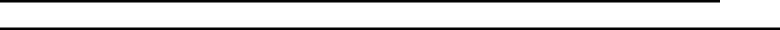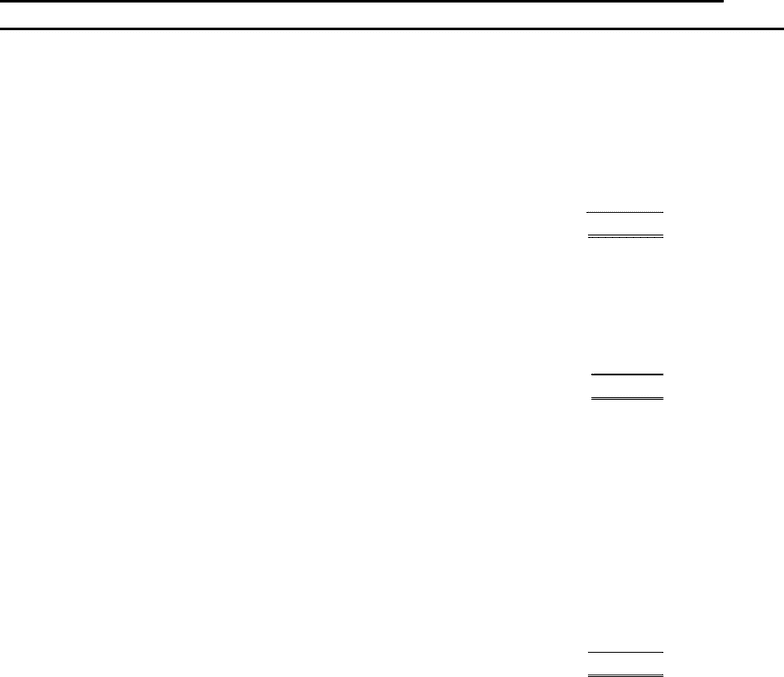Class Notes (1,000,000)
CA (620,000)
UTSC (30,000)
MGA (200)
Lecture 16

MGAB03H3 Lecture Notes - Lecture 16: Icq, Earnings Before Interest And Taxes, Weighted Arithmetic Mean

Department
Financial Accounting
Course Code
MGAB03H3
Professor
G.Quan Fun
Lecture
16

This preview shows pages 1-3. to view the full 11 pages of the document.
MANAGEMENT
ACCOUNTING
Chapter 16
IN-CLASS
QUESTIONS (ICQs)
SOLUTION

Only pages 1-3 are available for preview. Some parts have been intentionally blurred.ICQ #1
16.21 RI, ROI and EVA - Brannard Company
A. Residual income
= operating income – (rate of return x average assets)
= (\$2,000,000 - \$1,200,000 - \$200,000) – (15% x \$3,000,000)
= \$600,000 - \$450,000
= \$150,000
B. ROI
= operating income / average investment
= \$600,000/\$3,000,000
= 20%
C. EVA
= adjusted after-tax income – [weighted average cost of capital x (adjusted total
assets – current liabilities)]
= [\$600,000 x (1-0.36)] – [12% x (\$3,000,000 - \$200,000)]
= \$384,000 - \$336,000
= \$48,000

Only pages 1-3 are available for preview. Some parts have been intentionally blurred.ICQ #2
16.23 ROI, RI, Breakeven Point, Contribution Margin - Oslo Company
[Note: Part C of this problem requires knowledge of breakeven analysis from Chapter 3.]
A. Before calculating ROI, it is first necessary to calculate income:
Sales (300,000 * \$2) \$600,000
Variable costs (450,000)
Fixed costs (90,000)
Income \$ 60,000
ROI = \$60,000/[(\$500,000 + \$700,000)/2] = 10%
B. Residual income:
Income \$ 60,000
Minimum return [(\$500,000 + \$700,000)/2 x 0.15] (90,000)
Residual income \$(30,000)
C. Variable cost per unit: \$450,000/300,000 = \$1.50
Breakeven number of units:
Breakeven units = Fixed costs/ CM per unit
CM per unit = \$2.00 - \$1.50 = \$0.50
BE units = \$90,000 / \$.50
BE units = 180,000 units
D. Sales \$600,000
Variable costs 450,000
Contribution margin \$150,000